# The Dark Energy Survey

The Dark Energy SurveyDES logo

The Dark Energy Survey (DES) is a survey that aims to probe the dynamics of the expansion of the universe and the growth of large scale structure. The collaboration is composed of research institutes and universities from United States, Brazil, England, Germany and Spain. The survey will use the 4-meter Victor M. Blanco Telescope located at Cerro Tololo Inter-American Observatory (CTIO) in Chile, and the main innovation of that project consists in the development of a new camera which is commonly called DECam. This camera will allow astronomers to take more sensitive images in the red part of the visible spectrum and in the near infrared, in comparison to current equipment installed at Victor M. Blanco Telescope. In addition, DECam has one of the widest field of view available for ground-based optical and infrared images. According to the official website, its field is so large that a single image will record data from an area of the sky 20 times the size of the moon as seen from the earth. The survey will image 5000 degrees of the southern sky and will take five years to complete.

## Overview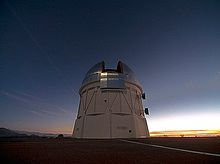The Victor M. Blanco Telescope, where the DECam will be installed.

The Dark Energy Survey will investigate the dynamics of the universe and the large-scale structure using four techniques. The first one consists in the observation of the light curve of Type Ia supernova. According to the accepted theory, a Type Ia supernova is an explosion of a white dwarf star that orbits around a companion star, caused by the accretion of mass from the companion star, which makes it unstable, starting, as a consequence, a gigantic thermonuclear explosion. For cosmology, these events are important because they are very bright, which allows astronomers to detect them at very large distance, and their luminosity distance (there are more than one definition of distance in cosmology and they are not equivalent. Consequently, it is important to specify what kind of distance you are measuring) can be inferred by the observation of their light curves. Finally, the standard model of cosmology, which is based on some assumptions that includes the validity of General Relativity and the large scale homogeneity and isotropy of our universe, predicts that astronomers can constrain the properties of the expansion of the universe based on the observation of the luminosity distance and the redshift from far away type IA supernova.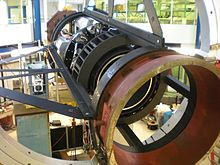Overview of the DECam structure

The other three techniques that Dark Energy Survey will use to constrain the properties of the expansion and the large scale structure of our universe are Baryon Acoustic Oscillations, Counts of Galaxy Cluster and Weak Lensing. In contrast to type IA supernova luminosity distance measures, these probes allow scientists to understand simultaneously the expansion of the universe and the evolution of the dark matter density field perturbations. These perturbations were indispensable to the formation of galaxies and galaxy clusters. Basically, the standard model of cosmology assumes that quantum fluctuations of the density field of the various components that were present when our universe was very young were, somehow, enhanced through a very rapid expansion called inflation. After that, gravitational collapse makes this initial fluctuation bigger and bigger, allowing baryons to fall into the gravitational potential field of more dense regions of space in order to form galaxies. Nevertheless the growth rate of these dark matter halos is sensitive to the dynamics of the expansion of the universe and the Dark Energy Survey will use this connection to probe the properties of that expansion.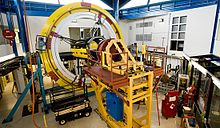Fermilab laboratory setting-up the DECam structure

The new camera that will be installed at the Victor M. Blanco Telescope by DES collaboration will bring new observational possibilities, which are not available for the current surveys, like, for instance, the Sloan Digital Sky Survey. One significant difference between the current CCD at the Victor M. Blanco Telescope and the DECam is the quantum efficiency in the red part of the visible spectra and in the near infrared. While the former has high quantum efficiency for blue light and a significant lower sensitivity for wavelengths located in the near infrared, the latter was designed to have its maximum sensitivity for red light. This is a very important property for the observation of very distant sources, like type IA supernovae or galaxy cluster, because the expansion of the universe redshift the photons emitted from a given source. On the other hand, Silicon, which is the main element used to make CCDs, becomes transparent for infrared light, and this issue made the development of the DECam's CCD a technological challenge.

The DES collaboration is led by Josh Frieman and composed of many research institutes and universities. Inside the United States, The Fermi National Accelerator Laboratory (Fermilab), the University of Chicago, the National Optical Astronomy Observatory, the Ohio State University, the Texas A&M University, the University of Illinois at Urbana-Champaign, the Lawrence Berkeley National Laboratory, the University of Michigan, the University of Pennsylvania, the Argonne National Laboratory, the University of California Santa Cruz, the SLAC National Accelerator Laboratory and the Stanford University are involved in this project. In addition, the Brazilian Center for Physics Research (Centro Brasileiro de Pesquisas Físicas - CBPF), the National Observatory (Observatório Nacional - ON) and the Federal University of Rio Grande do Sul (Universidade Federal do Rio Grande do Sul - UFRGS) are the Brazilian institutes involved.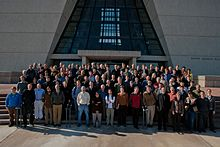Collaboration meeting of the Dark Energy Survey at Fermilab. October 2010

The British institutions that are participating in the DES collaboration are the University College London, the University of Cambridge, the University of Edinburgh, the University of Portsmouth, the University of Sussex and the University of Nottingham. Moreover, the Cluster for Excellence for Fundamental Physics and the Ludwig-Maximilians University of Munich (Ludwig-Maximilians Universität) are the German institutes affiliated. Finally, the Institute of Space Sciences (Instituto de Ciencias del Espacio), the Institute of High Energy Physics (Institut de Fisica d'Altes Energies) and the Center for Energy, Environment and Technology Research (Centro de Investigaciones Energeticas, Medioambientales y Tecnologicas - CIEMAT) are the Spanish institutes involved.

Finally, due to the complexity of the science involved in the Dark Energy Survey, the scientist that participate in this project were divided in many working groups. Currently the working groups are: the weak lensing working group, the clusters working group, the large scale structure working group, the supernova working group, the galaxy evolution working group and the Strong Lensing working group. There is also people working on simulations, observing and survey strategy, calibration, photometric redshift, data challenges, quasars and the mechanical, electronic and optical development of the DECam. Every year there is an annual meeting between all groups in order to release new results about the development of the project. Besides that, the collaboration has a website, where scientist can release new results, presentations and articles. Some of the releases in this website are open for the general public.

## DECam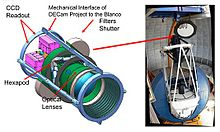Left: DECam Overview, Right: Blanco 4m telescope, red arrow point to prime focus cage where DECam will be mounted

DEcam is a large camera being built to replace the current prime focus camera on the Victor M. Blanco Telescope. The camera consists of three major components: optics, mechanics, and CCDs.

### Mechanics

The mechanics of the camera consists of a filter changer with an 8 filter capacity and shutter. There is also an optical barrel which supports 5 corrector lenses, the largest of which is 98 cm in diameter. These components are attached to the CCD focal plane which is cooled to -100 °C with liquid nitrogen in order to reduce thermal noise in the CCDs. The focal plane is also kept in an extremely low vacuum of 10−6 Torr to prevent the formation of condensation on the sensors. The entire camera with lenses, filters, and CCDs weighs approximately 4 tons. When mounted at the prime focus it will be supported with a hexapod system allowing for real time focal adjustment.

### Optics

In the current design the camera will be outfitted with g, r, i, z, y filters similar to those used in the SDSS survey. This will allow DES to get photometric redshift measurements to z~1, using the 4000 Å break for galaxies, a step-like spectral feature that occurs due to a number of absorption lines from ionized metals, and light curve fitting techniques for Type Ia supernova. DECam also contains 5 lenses acting as corrector optics to extend the telescope's field of view to a diameter of 2.2°.

### CCDs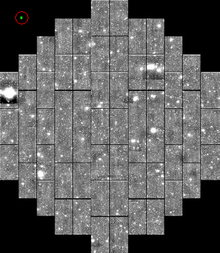Simulated image of the DECam CCD array at focal plane. Each large rectangle is a single CCD. The green rectangle in upper left corner represents the size of the iPhone 4 CCD relative to DECam CCDs

The scientific sensor array on DECam is an array of 62 2048x4096 pixel back-illuminated CCDs totaling 520 megapixels, there are an additional 12 2048x2048 pixel CCDs used for guiding the telescope, monitoring focus and alignment. The CCDs for DECam use a 15x15 micron pixel size, high resitivity silicon manufactured by Dalsa and LNBL. By comparison the OmniVision Technologies back-illuminated CCD that is currently employed in the iPhone 4 uses a 1.75x1.75 micron pixel size with 5 megapixels. The larger pixels allow DECam to collect more light per pixel improving low light sensitivity which is desirable for an astronomical instrument. DECam's CCDs also have a 250 micron crystal depth, this is significantly larger than most consumer CCDs. The additional crystal depth increases the path length travelled by entering photons. This in turn increases the probability of interaction and allows the CCDs to have an increased sensitivity to lower energy photons, extending the wavelength range to 1050 nm. From an astronomical point of view this is important because it allows you to look for objects at a higher redshift, increasing statistical power in the studies mentioned above. When placed in the telescope's focal plan each pixel has a width of 0.27“ on the sky, this results in a total field of view of 3 square degrees.

## Survey

The DES collaboration plans to complete a 5000 square degree survey in the southern sky spread out over 5 years. The survey is planned to reach a depth of 24th magnitude in i band over the entire area. The survey area was chosen to overlap with the survey area of the South Pole Telescope because its technique of finding clusters through the SZ effect compliments the optical techniques employed by DES. Within the 5000 square degree area there are 5 smaller patches totaling 30 square degrees which will use longer exposure times to search for Supernova.

## Supernovae

### Applications in Cosmology

Astrophysicists first discovered cosmic acceleration by examining the apparent brightness of tens of distant Type Ia supernovae, exploding stars that briefly become as bright as an entire galaxy of billions of stars. In current leading models of Type Ia supernovae, the explosions occur when a binary white dwarf star accretes matter from its companion star, becomes unstable (the mass limit when the star becomes unstable is still in dispute), and essentially is disrupted by a gigantic thermonuclear explosion. Although there are some variations, most of supernovae Ia follow a characteristic light curve—the graph of luminosity as a function of time—after the explosion with a maximum absolute magnitude of about -19.3. This homogeneity with the large brightness makes them one of the best standard candles to determine their distance.

To determine if the expansion rate of the universe is speeding up or slowing down over time, cosmologists make use of the finite velocity of light. It takes billions of years for light from a distant galaxy to reach the earth. Since the universe is expanding, the universe was smaller (galaxies were closer together) when light from a distant galaxy was emitted than it is today. If the expansion rate of the universe is speeding up due to dark energy, then the size of the universe increases more rapidly with time than if the expansion were slowing down. Using supernovae, we cannot quite measure the size of the universe versus time. Instead we can measure the size of the universe (at the time the star exploded) and the distance to the supernova. With the distance to the exploding supernova in hand, astronomers can use the value of the speed of light along with the theory of General Relativity to determine how long it took the light to reach the earth. This will then tell them the age of the universe when the supernova exploded.

### SN Data

To determine the distances to these stars, cosmologists use the fact that Type Ia supernovae are nearly "standard candles": exploding stars of this type all have nearly the same absolute brightness or luminosity when they reach their brightest phase. By comparing the apparent brightness of two supernovae (called as distance modulus), we can thus determine their relative distances.

mM = 5log 10dL + 25,

where m is the apparent brightness, M is the absolute/intrinsic brightness, and dL is the luminosity distance to the light source in units of megaparsecs (Mpc). This is similar to using the apparent brightness of a car’s headlights at night to estimate how far away it is: because light obeys an inverse-square law, a car 200 meters away from an observer will appear to have headlights four times dimmer than an identical car placed 100 meters away. Type Ia supernovae are the cosmic equivalent of cars with the same wattage of headlights.

To determine the second piece of the puzzle, the size of the universe at the time of explosion, astronomers measure the redshifts of supernovae from their known spectral lines and from the emission lines in the spectrum of host galaxies. When a supernova explodes, it emits light in the form of a wave. As the light wave travels towards the earth over billions of years, the universe continues to expand, stretching this traveling wave as it does. The more the universe has expanded between the explosion and when we see the light with our telescopes, the greater the increase in the wavelength of the light. The visible light with the longest wavelength is the colour red, so this process of increasing wavelength of the light wave is referred to as "redshifting". (For additional information on redshifts in DES, click here.)

According to Friedmann-Lemaître-Robertson-Walker metric, the luminosity distance in a flat universe, dL, at a given redshift depends on the composition of our Universe and its corresponding expansion history:$d_{L} = \frac{c}{H_0} (1+z) \int_0^z \frac{dz}{\sqrt{\Omega_M (1+z)^3 + \Omega_{DE} (1+z)^{3(1+w)} + \Omega_k (1+z)^2}}$

where dL is the luminosity distance, z is the redshift, c is the speed of light, H0 is the local expansion rate, ΩM is the matter content of the universe, ΩΛ is the dark energy content of the universe, Ωk due to curvature, and w is the dark energy equation of state parameter. For different models of Universe, we can thus infer the luminosity distance relationship with redshift respectively. Comparing the redshift with the distance for a large number of supernovae, we can derive the history of the cosmic expansion rate (see the Hubble diagram on the upper right). In 1998, such measurements were first reported for supernovae at large distances, those which exploded when the universe was only two-thirds its present size. These supernovae appeared about 25% fainter, that is, farther away, than expected, an effect attributed to the speed-up of cosmic expansion over the last several billion years.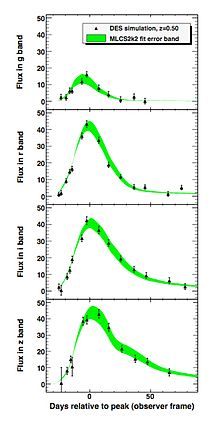Simulated DES supernovae light curves in four bands.

Controlling and calibration systematic effects are important for type Ia supernovae to be used as standard candles for cosmological analysis. One major issue that astronomers must wrestle with is the effect of dust extinction, the absorption of light by dust particles along the observational line of sight on the luminosity of the type Ia supernova. By analyzing measurements from a number of bands, as well as selecting regions of the sky for observation that are known to suffer from less dust extinction such as the poles of the Milky Way, the effects of dust can be understood.

### Supernovae Measurements in DES

A number of ambitious cosmological Supernova surveys, including the CFHT SNLS, ESSENCE, and SDSS-II SN, as well as several nearby searches have created a Hubble diagram out to redshift z ~ 1. Furthermore, searches using the Hubble Space Telescope are extending the SN Hubble diagram beyond z ~ 1. Upon these ground-based surveys, the Dark Energy Survey will continue this examination by discovering and making detailed measurements of several thousand supernovae with the aims of improving both the statistical precision of supernova cosmology and the control of systematic errors in using supernovae to measure distances. The Dark Energy Survey will measure the brightness of around 3,000 supernovae. These supernovae are billions of light years distant from earth. When the most distant ones DES will study exploded, the universe was only about half as big as it is now. The tentative plan for the Dark Energy Survey is for 30 square degrees of the sky to be observed in the time domain with two deep and eight shallow fields, leading to the discovery of approximately 6,000 supernovae, roughly two-thirds of which will have sufficient data from which distance measurements can be made.

## Baryon Acoustic Oscillations (BAO)

### Overview of Physics

Baryon Acoustic Oscillations (BAO) refer to oscillations within the baryon-photon plasma that filled the early universe. The presence of these oscillations imparted a characteristic signal in the density field of matter that can be seen today in the clustering of structure in the universe. The length scale at which this signal occurs throughout the universe can be used as a Standard ruler to constrain cosmology, and in particular, the evolution of dark energy. Measurements of BAO form a key part of the observation program of the Dark Energy Survey.

Before roughly 380,000 years after the big bang, the universe was a hot, dense plasma consisting almost entirely of photons, electrons and protons (the last two of these components are often referred to collectively as baryons, although technically this is a misnomer). The high temperature of the universe during this epoch prevented electrons and protons from combining to form neutral atoms. A consequence of the high degree of ionization of the universe was that baryons remained tightly coupled to photons through Thomson scattering. The tight coupling to photons created a source of pressure for the baryons, driving them apart, while the gravitational field of the plasma and dark matter acted to pull the baryons together. This competition between forces (pressure and gravity) enabled the photon-baryon plasma to support pressure waves which were sourced by the initial perturbations in the density field.

When recombination (cosmology) occurred at roughly 380,000 years after the big bang, the photons and baryons decoupled from one another and the plasma lost its ability to support sound waves. One consequence of this decoupling was that the oscillations in the photon-baryon plasma were imprinted on the photon fluid; these are the oscillations that we observe in the Cosmic Microwave Background (CMB) angular power spectrum. Another consequence of the decoupling was that the baryons lost their pressure support and began to collapse into gravitational potential wells. This somewhat abrupt change imparted a slight over-density of matter on the length scale given by the distance that sound waves could have traveled since the big bang, i.e. the sound horizon. In today’s universe, this length scale corresponds to roughly 100 Mpc, and the effect can be observed by looking for oscillations in the matter power spectrum, P(k).

### BAO as a Tool for Cosmology

Measurement of BAO are an important tool for probing cosmology, and in particular the evolution of dark energy. As described above, BAO imparts a characteristic signal in the matter power spectrum on the scale of the sound horizon at recombination. This signal in the power spectrum can be used as a standard ruler to map out the evolution of the Hubble parameter, H(z), and the angular diameter distance dA(z) with redshift. This in turn constrains the properties of dark energy and other cosmological parameters.

The angle subtended by a standard ruler as a function of redshift is related to the Hubble parameter, H(z) through the concept of the angular diameter distance. The angular diameter distance, dA, is defined as$d_A = \frac{\Delta \chi}{\Delta \theta}$

where Δχ is the physical extent of the standard ruler and Δθ is its observed angular extent. dA can also be expressed as an integral over H − 1(z):$d_A = \frac{1}{1+z} \int_0^z \frac{dz}{H(z)}$.

For the case of BAO, the physical size, Δχ, of the standard ruler (i.e. the sound horizon at recombination) can be constrained from the CMB-based measurements of ΩM and ΩB. As the equation above shows, by measuring the angle subtended by the standard ruler as a function of redshift, we are effectively measuring the integral of H − 1(z) over redshift. Furthermore, the redshift interval, Δz, extended by the standard ruler is directly related to the Hubble constant: Δz = H(z)Δχ. Thus, in principle we can also gain a direct measure of H(z) by measuring this redshift interval Δz. For the DES, however, the errors in the photometric redshifts will be too large to make a determination of H(z) in this way possible. Because dark energy affects the expansion history of the universe, measuring H(z) can help to constrain the properties of this mysterious component of the universe.

The steps needed to constrain cosmology from BAO observations are therefore :

1. Conduct a survey of tracers of the matter density field (e.g. galaxies)
2. Compute the power spectrum of the matter density field at different redshifts from these observations
3. Measure the angle subtended by the BAO sound horizon feature in the matter power spectrum at different redshifts (and, if possible, the redshift interval associated with the sound horizon)
4. Compute dA(z) (and thus the integral of 1 / H(z) and use to constrain cosmology

One important caveat relating to the first step above is that the survey must be large enough to actually encompass the sound horizon scale. As mentioned above, this scale corresponds to distance of roughly 100 Mpc today. Early surveys such as the CfA2 redshift survey covered volumes too small to make significant detections of the BAO feature. More recent surveys, such as the Sloan Digital Sky Survey, and future surveys, such as DES, cover sufficient volume to make the measurement possible.

There are a number of qualities that make BAO a useful tool for probing cosmology. For one, the ideas behind the technique are simple: there is a straightforward relationship between the physical size of the BAO feature, its observed angular size, and the cosmological terms. Secondly, doing cosmology with BAO requires only a large volume survey. Such surveys have been common in astronomy for years. Finally, BAO provides test of cosmology that is independent of other constraints.

There are also a number of significant challenges inherent to the BAO observation program. For one, measurements of BAO at redshifts less than about 0.3 are impossible because the volume contained within this redshift is too small to fully sample the BAO feature. Another complication is that the BAO feature in the power spectrum is affected by non-linear evolution of the matter density field. As structure in the universe grows, it causes a widening of the BAO feature in the power spectrum, and an increase of power on smaller scales. These affects must be accounted for when using BAO to constrain cosmology. BAO observations are also complicated by the fact that the common tracers of the matter density field such as galaxies are biased: they tend to live in places where the matter density is highest. This bias must be taken into account in order for BAO to be used to constrain cosmology. Despite these complications, BAO remains an attractive tool for cosmology.

### BAO Observations in DES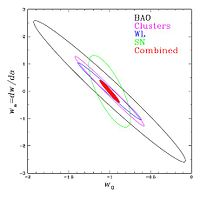68% CL forecast DES constraints in the w0wa plane from the four probes: BAO, clusters, weak lensing and SNe, each combined with the Planck CMB prior; the filled red region shows the constraints from combining the four methods.

The sample of 300 million galaxies with accurate photometric redshifts, Δz ~ 0.08, up to z ~ 1.4 provided by DES is well suited for measurement of BAO to study dark energy. The survey volume is 20 times that of SDSS photometric LRGs, enabling much higher precision measurements over a much wider redshift range. DES will measure the clustering on the sky of hundreds of millions of galaxies at different distances from us. These measurements will determine the angular scale of the sound horizon for galaxies at different redshifts. Combining those measurements together will provide information on the history of the cosmic expansion rate that complements the Type Ia supernova measurements. Defining a pivot epoch, ap, at which the uncertainty in the dark energy equation of state w(a) is minimized for a given measurement, the figure of merit (FoM), which is proportional to the reciprocal of the area in the w0wa plane that encloses the 95% CL region, is${[\sigma{(w_{p})}\sigma{(w_{a})}]}^{-1}$. Based on the fiducial cosmological model and some assumptions, the constrains of parameters can be calculated and confined.

The main theoretical uncertainties in the interpretation of BAO measurements are the effects of nonlinear gravitational evolution and of scale-dependent bias between galaxies and dark matter. Non-linear evolution erases the acoustic oscillations on small scales, while both non-linear mode coupling and scale-dependent bias can shift the positions of the BAO features. Scale-dependent bias on large scales is potentially more of a concern for interpreting the broad-band power spectrum shape than for the BAO signal. Simulations that will become available over the next few years, should enable us to calculate the corrections due to non-linearity and scale-dependent bias to sufficient accuracy that the remaining systematic uncertainty will be small compared to the DES statistical errors on large scales. In addition, since the bispectrum(The Fourier transform of the second-order cumulant used to search for nonlinear interactions) responds to non-linearity and bias differently from the power spectrum, measurement of the angular bispectrum shape in DES will provide a cross-check on these effects, constrain this scale-dependence and determine the efficacy and robustness of including the broad-band shape in constraining dark energy.

BAO are in principle sensitive to the uncertainty in the variance, Δσz , and in the bias, Δzbias , of the photo-z estimates in redshift bins. To ensure that the dark energy parameter constraints are degraded (i.e., the errors increased) by no more than 10%, these uncertainties in z and zbias per redshift bin of 0.1 must be kept below ~ 0.01 (for w0) and ~ 0.005 (for wa). These performance levels should be conserved in DES, and the BAO constraints are therefore expected to be quite insensitive to uncertainties in photo-z parameters.

Photometric zero-point drift affects the number density of galaxies in each redshift bin that lie above the detection threshold. The DES survey strategy, with its multiple overlapping tiles, is designed to minimize photometric drifts and to make them negligible on the scale of the DECam field of view. In addition to direct control from the tiling strategy, DES have a number of internal cross-checks on such drifts, including the redshift evolution of the BAO and matter-radiation scales, cross-correlations between different photo-z bins, consistency with the angular bispectrum, and comparison of the power spectrum shape and features for different galaxy-type subsamples.

## Galaxy Cluster Counts

An important experimental probe of dark enery comes from counting galaxy clusters. The idea is simple: we can use cosmological models (theories) calculate how many galaxy clusters should be visible, then we can use telescopes to count how many galaxy clusters we actually see. Thus comparing how galaxy clusters are distributed in mass and redshift to predictions made by cosmological models, we can test these cosmological models.

The number of galaxy clusters that form depends on dark energy in two ways. First, dark energy influences how the universe expands, so it affects how the volume grows over time. Second, the formation of a galaxy cluster depends upon the interplay between gravity and dark energy. By measuring how the number of clusters grows over time, DES will probe the relative strengths of these two forces. In this method, DES scientists will measure the abundance of clusters at different times in the past by measuring the number of clusters with a particular redshift. DES will count some galaxy clusters so far away that the light the DES camera sees from them today left the clusters when the universe was less than half its current size! (z~1.3)

Unlike the supernovae and BAO methods, which are only sensitive to cosmic distances and thereby to the expansion rate, galaxy clusters probe both distances and the rate of growth of structure in the universe. By comparing results between these two different classes of probes, cosmologists can determine whether the current theory of gravity, Einstein's General Relativity Theory, is sufficient to explain cosmic acceleration.

### Predicting Number Counts of Galaxy Clusters from Theory

In this section we work through how cosmological models predict the number of observable galaxy clusters. For any given cosmological model, we can predict the proper number density of galaxy clusters as a function of redshift, nprop(z). Here, 'proper number desnity' means the number of galaxy clusters in one unit of proper volume. Refer to comoving distance for a discussion of proper distances. Thus by calculating the proper volume element as a function of redshift, d2Vprop, we can predict the number of galaxy clusters per unit redshift and solid angle. Predicting nprop(z) depends on the specific cosmological model, and beyond the scope of this Wikipedia article.

To calculate the proper volume element, we first calculate the proper area (dA) for a given redshift (z), radial coordinate (r) and solid angle (dΩ). We then calculate the distance (dl) in the redshift interval (z,z + dz). The proper volume is then$d^2V_{prop} = dA\ dl$

Using the FLRW metric, the proper area dA is given by$dA = a(t_e)r\ d\theta a(t_e) r \sin(\theta) d \phi = a_e^2 r^2 d\Omega = \frac{a_0^2 r^2 d\Omega}{(1+z)^2}$

where a(t) is the scale factor.

The distance dl in the redshift interval (z,z + dz) is equal to the distance light travels in an infinitellimal time step dt,$dl = c\ dt$

Relating this to the redshift z, we find$dl=\frac{da}{\dot{a}} = \frac{dz}{(1+z)} \frac{a}{\dot{a}} = \frac{dz}{H(z)(1+z)}$

where H(z) is the Hubble parameter:$H(z) = H_0 \sqrt{ \sum_i \Omega_i (1+z)^{3(1-w_i)} + \Omega_k (1+z)^2}$

for constant wi.

The proper volume element is then just the product of dA and dl:$d^2V_{prop} = dA\ dl = \frac{a_0^2 r^2(z)}{H(z)(1+z)^3} dl dz$

Thus when combined with the theoretically predicted proper number density of galaxy clusters nprop(z), the number of galaxy clusters per unit redshift per unit solid angle is:$\frac{d^2N}{dz d\Omega} = n_{prop}(z) \frac{d^2V_{prop}}{dz d\Omega} = \frac{n_{prop}(z) a_0^2 r^2(z)}{H(z) (1+z)^3}$

### Calculating Experimental Constraints

The Dark Energy Survey will measure the differential number of galaxy clusters, d2N / dzdΩ, as a function of redshift z. Thus by comparing these measurements with predictions made by cosmological models, we will be able to constrain possible values for the equation of state, w, for dark energy.

Specifically, we calculate d2N / dzdΩ using the following relation:$\frac{d^2N(z)}{dz d\Omega} = \frac{r^2(z)}{H(z)} \int_0^{\infty}{f(\mathcal{O},z)d\mathcal{O}} \int_0^{\infty}{p(\mathcal{O}\vert M,z) \frac{dn(z)}{dM} dM}$

The first piece,$f(\mathcal{O},z)$, is the 'selection function' for the observable quantity$\mathcal{O}$. Examples of observable quantities are optical richness, X-ray luminosity, SZE flux, and weak lensing mass. In the Dark Energy Survey, different observable quantities will be used in different cluster finders.

The second function,$p(\mathcal{O}\vert M,z)$, is called the 'mass-observable relation'. This function gives the probability that a galaxy cluster with mass M and redshift z will have a particular value fo the observable$\mathcal{O}$.

Finally, the function dn(z) / dM is the 'theoretical mass function'. This function is used to calculate the expected number of galaxy clusters with a mass M at redshift z. This theoretical mass function encodes how the number of galaxy clusters depends on dark energy.

### Cluster Finding

To constrain cosmological models with galaxy clusters, we must have a very large number of galaxy clusters. As discussed above, we also need a well-known selection function, a theoretical mass function and a mass-observable relation.

The dark energy survey will discover a large number of galaxy clusters. The effectiveness of DES at finding clusters is due to its state-of-the-art camera, which will measure clusters out to a very faint magnitude, and the strategy of observing a large area of sky. The DES is sensitive to galaxy clusters that have masses greater than 5 * 1013Msun and contain > 10 bright red-sequence galaxies. By looking out to z = 1.5 (assuming ΛCDM cosmology), the DES expects to detect about 170,000 galaxy clusters.

To search for galaxy clusters in the optical data, one technique DES will use is a technique known as red sequence optical cluster finding. This well-known technique has been applied to smaller cluster samples from the SDSS and the RCS-II cluster surveys. The difficulty lies in calibrating the mass-observable relation for clusters and defining the selection function (purity and completeness) for the cluster finders.

The largest systematic uncertainty in using galaxy clusters to study cosmology comes from the mass-observable relation. Theories predict galaxy cluster masses, while experiments measure different observable quantities (i.e. optical richness) for galaxy clusters. In order to constrain cosmological models we must be able to allow the halos to evolve in time in order to make predictions for what we should see today. These simulations are used to predict mass-observable relations for the DES.

The self-calibration method takes advantage of the fact that the shape and amplitude of the cluster abundance function, dn / dz, can be measured. On the theory side dn / dz also depends on the mass-observable relation and the selection function. By checking for consistency between measurements of dn / dz and these functions, the self-calibration method provides an important double-check of systematic errors.

Finally, the mass-observable relation for the DES will be calibrated directly using weak gravitational lensing. Conceptually, by measuring the cosmic shear the weak lensing method can provide unbiased estimates of the virial mass for a sample of clusters. In practice, in order to calibrate the mass-observable relation for a specific observable (i.e. cluster richness) DES clusters will be binned by that observable and redshift. For each of these bins, the average cluster mass profile will be calculated using weak lensing. Relating the observable quantity to the calculated cluster mass profile produces the mass-observable relation.

To address the selection function (purity and completeness of the optically selected sample), the DES collaboration has developed a number of different cluster finding algorithms and tested them on mock catalogs. These mock catalogs are produced by the simulations mentioned above, and are then mapped into full sky simulations. The cluster finding algorithms are then run on these simulations to 're-find' the simulated galaxy clusters. Because the original simulated cluster distribution is known, this procedure tests how well the cluster finding algorithms work.

## Weak Lensing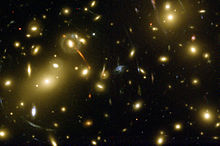Strong lensing in cluster Abell 2218. Credit: NASA/ESA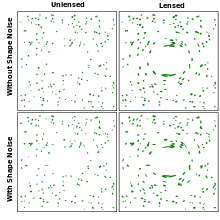The plots on the right show the effects of gravitational lensing on circular (top) and elliptical (bottom) galaxies in the plots to the left. Distortion shown here is greatly exaggerated relative to real astronomical systems.

Gravitational lensing occurs when light from distant sources such as quasars or galaxies is bent by the gravitational field of a massive object. An image of a Abell 2218, a cluster of galaxies, shows how the intervening matter field distorts the light from background galaxies. The effect around the cluster is so strong, that multiple images of the source galaxy are seen as arcs. This is referred to as strong gravitational lensing.

The history of growth of large scale structure (LSS) can give us a handle on the interplay between gravity and dark energy. However, most of this structure is made up of dark matter, which cannot be detected by standard astronomical means. The cosmological gravitational field can also bend the light from distant sources but in this case the images of galaxies are distorted, stretched and magnified, in small amounts. This is referred to as weak gravitational lensing.

This small distortion of the image of a galaxies referred to as cosmic shear and can amount to a typical stretching of an image on the order of 2 percent. The effect is too small to be measured for an individual galaxy. Fortunately, the same matter density field affects many galaxies in the same part of the sky and by studying a large number of galaxies in the same area of the sky, astronomers can look for alignments in cosmic shear statistically.

This is achieved by measuring the shear-shear correlation function, a two point function, or its Fourier Transform, the shear power spectrum. DES will measure the shear power spectrum as a function of photometric redshift. Another statistic that can be used is the angular correlation function between the foreground galaxy positions and the shear of the source galaxy, the so called galaxy-shear correlation.

Since shear is sensitive to the matter density field, which is dominated by dark matter, it is less sensitive to baryonic effects, although such effects may have a large enough contribution to the evolution of the matter power spectrum at small scales, such that we can no longer distinguish between predictions of interesting dark energy models. The calculation of the non-linear matter power spectrum poses another challenge for weak lensing measurements and must include baryonic effects.

DES will be able to probe Dark Energy because cosmic shear measurements are sensitive to the evolution of the matter power spectrum (linear growth of structure) and the distance-redshift relation (expansion history and geometry). Higher order statistics such as the three-point function or the bispectrum, which combine measurements of the CMBR anisotropy and galaxy distribution will be able to break degeneracies between geometry, growth of structure and spatial curvature.

### Systematics

The ability of DES to observe cosmic shear is limited by the systematics of the telescope, the number of galaxies observed, and the intrinsic correlation between ellipse directions of galaxies. The primary systematics of the telescope are described by the point spread function of the telescope. Distortions in the point spread function caused by the mirror, optics, or geometry of the various telescope components will act to produce a false cosmic shear image. These distortions can be caused by wind, thermal contraction, misalignment, or a variety of other effects. Fortunately, most of these effects can be corrected for by actively measuring the point spread function of the telescope. The point spread function of the telescope will be repeatedly measured by observing stars within our own galaxy. When the telescope observes these stars they should look like point sources, if they appear as extended streaks instead of points you can record the shape and direction of the streak and then correct out for this effect.

Since cosmic shear cannot be measured for a single galaxy and can only be detected statistically from many galaxies, the level to which cosmic shear can be measured depends on the amount of available galaxies being lensed. If a large number of galaxies with intrinsically random oriented ellipse directions can be observed in the same region of the sky, there will be less of an error on the final measurement for the cosmic shear. However, if there exists an intrinsic ellipse direction for a particular group of galaxies in a region of the sky, it can yield an artificially high value for the cosmic shear in that region.

## Data Management

The survey's data are to be processed by the Dark Energy Survey Data Management System, which is centered at the National Center for Super Computing Applications at the University of Illinois at Urbana Champaign. The DES will release the survey's raw and reduced DECAM images after a one year proprietary period, via its portals at NCSA. The DESDM will also make two full releases of its data products, one approximately mid-way though the survey, and a final release at the end of the survey.

## Footnotes

1. ^ DES Collaboration Page, DES Collaborators.
2. ^ a b DES-Brazil, DES-Brazil Consortium.
3. ^ a b DES Collaboration Page - Camera, DECam.
4. ^ The Victor M. Blanco Telescope, The Victor M. Blanco Telescope official website.
5. ^ News about Flash Code News about the first 3D successful simulation of a type IA supernova.
6. ^ a b DECam Presentation, Pdf Presentation about the specific details about how a CCD device works and about the specific properties of the DECam, made by a Fermilab specialist.
7. ^ The Project - The Dark Energy Survey Collaboration, The DES Project Site.
8. ^ SDSS FIlter Description
9. ^ a b Adam G. Riess et al. (Supernova Search Team) (1998). "Observational evidence from supernovae for an accelerating universe and a cosmological constant". Astronomical J. 116 (3): 1009–38. arXiv:astro-ph/9805201. Bibcode 1998AJ....116.1009R. doi:10.1086/300499.
10. ^ a b http://mwhite.berkeley.edu/BAO/bao_iucca.pdf
11. ^ Gladders, M.D., et al., 2007, ApJ, 655 (1): 128–134.
12. ^ Gladders, M. D., et al. 2006, astro-ph/0603588
13. ^ DES Collaboration Page - Weak Lensing, Weak Lensing.
14. ^ https://www.darkenergysurvey.org/reports/proposal-standalone.pdf
15. ^ https://www.darkenergysurvey.org/the-project/survey_documents/DES-DETF/Supplements_DES-DETF_v1.6.pdf
16. ^ a b https://www.darkenergysurvey.org/the-project/survey_documents/DES-DETF/weinberg.pdf

Wikimedia Foundation. 2010.

### Look at other dictionaries:

• Dark energy — Not to be confused with dark matter, dark fluid, or dark flow. Physical cosmology …   Wikipedia

• Dark Energy Space Telescope — The Dark Energy Space Telescope (Destiny), was a planned project by NASA and DOE, designed to perform precision measurements of the universe to provide an understanding of dark energy. The space observatory will derive the expansion of the… …   Wikipedia

• dark energy — noun A hypothetical form of energy which, it is supposed, is spread uniformly throughout space (and time) and has anti gravitational properties: it represents a possible mechanism for the cosmological constant, and thus is one of the possible… …   Wiktionary

• Out of the Dark (2010 novel) — Out of the Dark   …   Wikipedia

• Dark matter — Not to be confused with antimatter, dark energy, dark fluid or dark flow. For other uses, see Dark Matter (disambiguation) …   Wikipedia

• Dark fermentation — is the fermentative conversion of organic substrate to biohydrogen. It is a complex process manifested by diverse group of bacteria by a series of biochemical reactions involving three steps similar to anaerobic conversion. Dark fermentation… …   Wikipedia

• Dark-sky movement — The dark sky movement is a campaign by people who want to reduce light pollution so people can see the stars, to reduce the effects of unnatural lighting on the environment, and to cut down on energy usage. The movement started with professional… …   Wikipedia

• The Wash — is the square mouthed estuary on the northwest margin of East Anglia on the east coast of England, where Norfolk meets Lincolnshire. It is among the largest estuaries in the United Kingdom. It is fed by the Rivers Witham, Welland, Nene and Great… …   Wikipedia

• The Man in the High Castle —   …   Wikipedia

• Dark galaxy — A dark galaxy is a hypothetical galaxy composed of dark matter. Dark galaxies receive their name because they have no detectable stars and are theoretically invisible. An influential community of scientists conjecture the existence of dark… …   Wikipedia